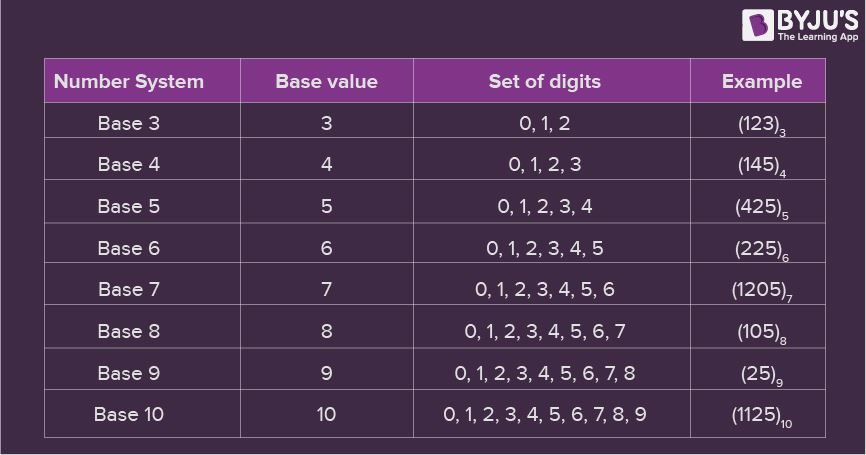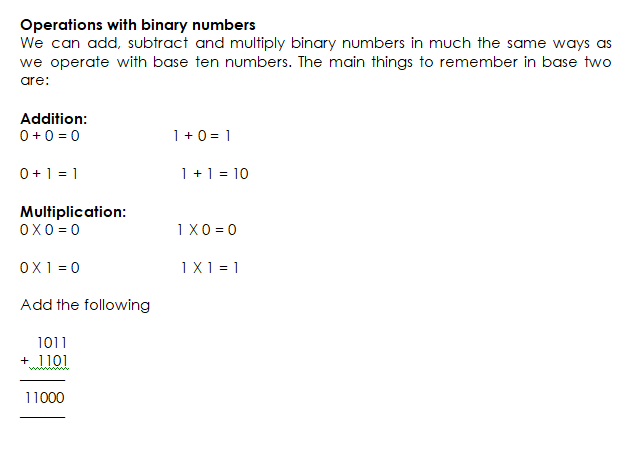# Overview of Number Bases II

Welcome to class!

In today’s class, we will be talking more about the overview of number bases. Enjoy the class!

### Overview of Number Bases IIThere are some other binary-related terms you’ll need to know. Firstly, a bit is a binary digit – i.e. a single occurrence of 0 or 1. This is the smallest unit of storage you can have inside a computer. Groups of 8 bits are called bytes. A byte can be used to represent a number, or colour, or a character (e.g. using ASCII). You may also hear the term nibble, which is 4 bits. Finally, a word is the largest numbers of bits that a processor can handle in one go – for example, when we say that new computers have 64-bit processors, we mean that the word length is 64-bits or 8 bytes.

The largest value that you can store using a particular number of bits can be determined quite easily. Using n bits, the largest value you can store is 2n – 1, and the number of different values you can store is 2n (from 1 to 2n – 1, and then 0 as well). So using 8 bits, the largest number you can store is 28 – 1 = 255, and the number of possible values is 28 = 256 (i.e. 0 – 255). A 32-bit computer can therefore handle values up to 4,194,967,296 in one clock cycle – it can cope with larger numbers, but it would need to split them up first.

#### Octal (Base 8):

I’ve never come across anything that uses octal! I think it’s probably included on exam specifications for purely academic reasons, and because it’s easy to convert into binary (see below).

Hexadecimal is still used quite a lot – particularly for things like colours in HTML or programming languages. It’s also quite useful because representations of large numbers are relatively compact, but are easily converted to binary so that you can see the bit patterns.

##### Shifting Bits

You’ve no doubt noticed that with numbers in base 10, you can move the digits left or right one place by multiplying or dividing the number by 10. The same trick works with different number bases – you just multiply and divide by the base number (e.g. multiply by 2 in binary to shift the bits left one place).

This can be useful for things like creating hexadecimal colour values (e.g. for web pages). In a 24-bit system (such as HTML), colours are represented by 24-bit numbers from 000000 to FFFFFF (each hexadecimal digit corresponds to 4 bits – see below). The 24 bits are made up of 8 bits each for the amount of red, green and blue in the colour.

So, each component is represented by 8 bits – i.e. a number from 0 to 255. If you know how much red, green and blue you want, how do you combine them to find the complete colour? For HTML, the correct order of the bits is RRGGBB (r = red, g = green, b = blue), so what we need to do is “shift” the values of green and red components, and then add all three components together.

We can leave the blue value as it is, but we need to move the green value along two places. To move along one place in hexadecimal, we multiply by 16, so to move along two places, just do it twice – 16 x 16 = 256 – so multiply the green value by 256. For the red value, we need to move four places – 16 x 16 x 16 x 16 = 65,536 – so we multiply the value of the red component by 65,536.

If you were just trying to work out the colour yourself, you wouldn’t need to go through these steps, but if you were to create a program like my colour mixer, then this is how you’d do it.

#### Binary:

Let’s look at base-two, or binary, numbers. How would you write, for instance, 1210 (“twelve, base ten”) as a binary number? You would have to convert to base-two columns, the analogue of base-ten columns. In base ten, you have columns or “places” for 100 = 1, 101 = 10, 10= 100, 103 = 1000, and so forth. Similarly in base two, you have columns or “places” for 20 = 1, 21 = 2, 22 = 4, 23 = 8, 24 = 16, and so forth.

The first column in base-two math is the units column. But only “0” or “1” can go in the units column. When you get to “two”, you find that there is no single solitary digit that stands for “two” in base-two math. Instead, you put a “1” in the twos column and a “0” in the units column, indicating “1 two and 0 ones”. The base-ten “two” (210) is written in binary as 102.

A “three” in base two is actually “1 two and 1 one”, so it is written as 112. “Four” is two-times-two, so we zero out the twos column and the units column, and put a “1” in the fours column; 410 is written in binary form as 1002. Here is a listing of the first few numbers:

 decimal (base 10) binary (base 2) 0 1 2 3 4 5 6 7 8 9 10 11 12 13 14 15 16 0 1 10 11 100 101 110 111 1000 1001 1010 1011 1100 1101 1110 1111 10000 0 ones 1 one 1 two and zero ones 1 two and 1 one 1 four, 0 twos, and 0 ones 1 four, 0 twos, and 1 one 1 four, 1 two, and 0 ones 1 four, 1 two, and 1 one 1 eight, 0 fours, 0 twos, and 0 ones 1 eight, 0 fours, 0 twos, and 1 one 1 eight, 0 fours, 1 two, and 0 ones 1 eight, 0 fours, 1 two, and 1 one 1 eight, 1 four, 0 twos, and 0 ones 1 eight, 1 four, 0 twos, and 1 one 1 eight, 1 four, 1 two, and 0 ones 1 eight, 1 four, 1 two, and 1 one 1 sixteen, 0 eights, 0 fours, 0 twos, and 0 ones

Converting between binary and decimal numbers is fairly simple, as long as you remember that each digit in the binary number represents a power of two.

Convert 1011001012 to the corresponding base-ten number.

I will list the digits in order, and count them off from the RIGHT, starting with zero:

The first row above (labelled “digits”) contains the digits from the binary number; the second row (labelled” numbering”) contains the power of 2 (the base) corresponding to each digits. I will use this listing to convert each digit to the power of two that it represents:

1×28 + 0×27 + 1×26 + 1×25 + 0×24 + 0×23 + 1×22 + 0×21 + 1×20

= 1×256 + 0×128 + 1×64 + 1×32 + 0×16 + 0×8 + 1×4 + 0×2 + 1×1

= 256 + 64 + 32 + 4 + 1

Then 1011001012 converts to 35710

##### Operations with binary numbers

We can add, subtract and multiply binary numbers in much the same ways as we operate with base ten numbers. The main things to remember in base two are:

0 + 0 = 0                      1 + 0 = 1

0 + 1 = 1                        1 + 1 = 10

Multiplication:

0 X 0 = 0                       1 X 0 = 0

0 X 1 = 0                        1 X 1 = 1

1011

+  1101

——

11000

———###### Evaluation
1. (1010.011)2= (a) (10.365)10   (b) (10.375)10   (c) (11.365)10    (d) (11.375)10
2. (41)⊂10 in binary is (a) 101101   (b) 101011  (c) 101001  (d) 101101
3. Convert (0.6875)⊂10 to binary (a) 0.1011  (b) 0.1011  (c) 0.0101  (d) 0.0111
4. Convert (153.513)10in octal number system is(a) 231.408517  (b) 231.407517  (c) 231.406517  (d) 231.406617
5. All these are different types of number systems except (a) Arabic  (b) Babylonian  (c) Roman  (d) Nigerian

In our next class, we will be talking about Data Representation.  We hope you enjoyed the class.

Should you have any further question, feel free to ask in the comment section below and trust us to respond as soon as possible.

How Can We Make ClassNotesNG Better - CLICK to Tell Us💃